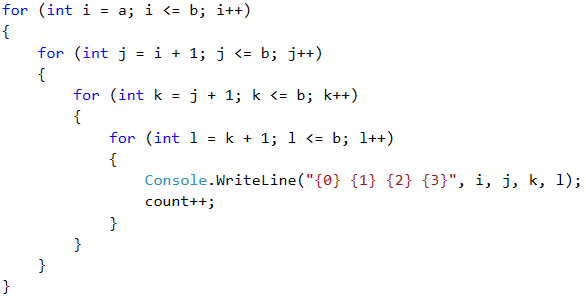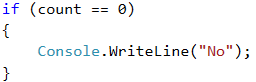# Problem: Increasing 4 Numbers

The next sample exam problem is about using nested loops and program logic to generate all possible combinations of 4 increasing numbers in given range.

## Video: Increasing 4 Numbers

Watch the video lesson about solving the "Increasing 4 Numbers" problem: https://youtu.be/2DuNHqmbP5Y.

## Problem Description

For given pair of numbers a and b generate all four number n1, n2, n3, n4, for which a ≤ n1 < n2 < n3 < n4 ≤ b.

In combinatorics such a selection of subset from given set (or range) is called "combination", so the problem is essence is to generate all combinations of 4 elements from given range of integers.

## Input

The input contains two integers a and b in the range [0 … 1000], one per line.

## Output

The output contains all numbers in batches of four, in ascending order, one per line.

## Sample Input and Output

Input Output Input Output
3
7
3 4 5 6
3 4 5 7
3 4 6 7
3 5 6 7
4 5 6 7
15
20
15 16 17 18
15 16 17 19
15 16 17 20
15 16 18 19
15 16 18 20
15 16 19 20
15 17 18 19
15 17 18 20
15 17 19 20
15 18 19 20
16 17 18 19
16 17 18 20
16 17 19 20
16 18 19 20
17 18 19 20
Input Output Input Output
5
7
No 10
13
10 11 12 13

We will read the input data from the console. We also create the additional variable count, which will keep track of existing number ranges.## Implementation with 2 Numbers

We will most easily solve the problem if we logically divide it in parts. If we are required to draw all the rows from a number between a and b, we will do it using one loop that takes all the numbers from a to b. Let's think how to do this with series of two numbers. The answer is easy – we will use nested loops.We can test the incomplete program to see if it's accurate so far. It must print all pairs of numbers i, j for which i ≤ j.

Since each next number of the row must be greater than the previous one, the second loop will run around i + 1 (the next greater number). Accordingly, if there is no sequence of two incremental numbers (a and b are equal), the second loop will not be fulfilled, and nothing will be printed on the console.

## Implementation with 4 Numbers

Similarly, what remains is to implement the nested loops for four numbers. We will add an increase of the counter that we initialized in order to know if there is such a sequence.Finally, we will check if the counter is equal to 0 and we will print "No" on the console accordingly, if so.